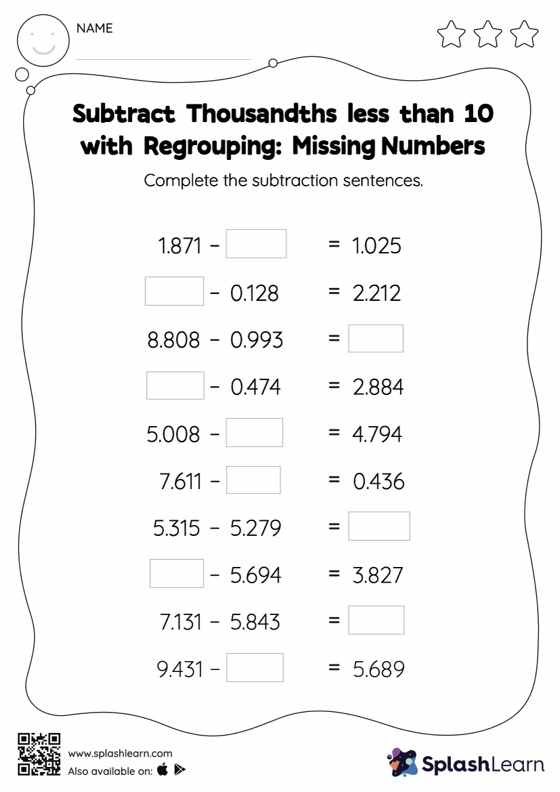# Subtract Thousandths less than 10 with Regrouping: Missing Numbers Worksheet

Home > Subtract Thousandths less than 10 with Regrouping: Missing NumbersHelp your little one develop a knack for math with this subtract thousandths less than 10 with regrouping worksheet. Students find the missing number while subtracting decimals by using the relationship between addition and subtraction. To reach the answer, they also use the relationship between ones, tenths, hundredths, thousandths, etc., To regroup the numbers in subtract thousandths less than 10 with regrouping worksheet. In each problem, the numbers are laid out in the horizontal format. Students should try to use different strategies involving composing and decomposing numbers to solve these problems. This will help them develop flexibility and fluency.